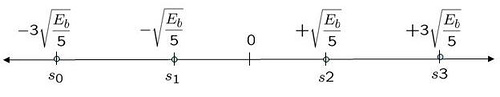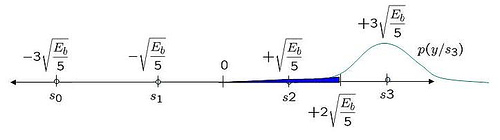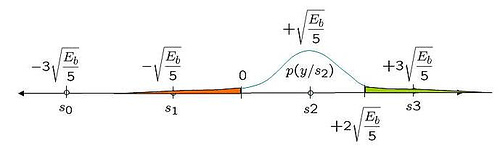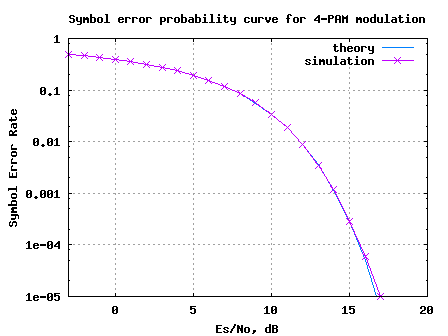- DSP log - http://www.dsplog.com -

Symbol Error Rate (SER) for 4-PAM

Posted By Krishna Sankar On October 7, 2007 @ 8:21 pm In Modulation | 42 Comments

Following discussion of bit error rate (BER) for BPSK  and bit error rate for FSK , it may be interesting to move on to discuss a higher order constellation such as Pulse Amplitude Modulation (PAM).

Consider that the alphabets used for a 4-PAM is (Refer example 5-34 in [DIG-COMM-BARRY-LEE-MESSERSCHMITT]).

The average energy of the constellation assuming all the alphabets are equally likely is,

.

The constellation plot for a 4-PAM signal after normalization can be as shown below.Figure: Constellation plot for 4 PAM modulation

## Channel Model

Now comes the interesting task – analysis of symbol error probability for the modulation scheme in additive white Gaussian noise condition. The noise follows the Gaussian probability distribution function, with and .

## Computing the symbol error rate

Using the derivation provided in Section 5.2.1 of [COMM-PROAKIS]  as reference:

or or or .

Let us first consider the case was transmitted.

The conditional probability distribution function (PDF) of given was transmitted is :

.Figure: Probability distribution function when the alphabet +3 is sent

Using midway point between +1 and +3 as the detection threshold, i.e. for received signal .

With this threshold, the probability of error given is transmitted is (the area in blue region):

.

Note: The complementary error function, .

Given that the constellation of +3 and -3 is symmetric, it is reasonably intuitive that the probability of error given is transmitted is also,

.

Case where is transmitted

The conditional probability distribution function (PDF) of given was transmitted is :

.Figure: Probability distribution function when the alphabet +1 is sent

The probability of error given is transmitted is (the area in green and red region):

Given that the constellation for +1 and -1 is symmetric, it is reasonably intuitive that the probability of error given is transmitted is also,

.

Total probability of symbol error

Given that all the alphabets are equally likely, the total probability of symbol error is,

.

Assuming, and , are equally probable i.e. , the symbol error probability is,

.

## Simulation Model

The Matlab/Octave script for generating a 4-PAM transmission, pass it through additive white Gaussian noise and demoduation at the receiver will be useful for understanding the concept further. The symbol error rate plots obtained from simulations compare well with the theoretical derivations.Figure: Symbol Error Rate for 4-PAM modulation

## Reference

[DIG-COMM-BARRY-LEE-MESSERSCHMITT]Digital Communication: Third Edition, by John R. Barry, Edward A. Lee, David G. MesserschmittURL to article: http://www.dsplog.com/2007/10/07/symbol-error-rate-for-pam/

URLs in this post:

 bit error rate (BER) for BPSK: http://www.dsplog.com/2007/08/05/bit-error-probability-for-bpsk-modulation/

 bit error rate for FSK: http://www.dsplog.com/2007/08/30/bit-error-rate-for-frequency-shift-keying-with-coherent-demodulation/